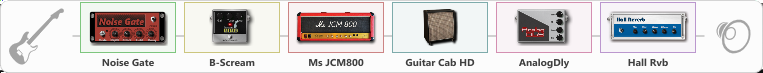# Knocking Solo

Discussion in 'ToneLib-GFX presets' started by Janusz K, Apr 24, 2022.

1. Knocking Solo

Preset name: Knocking Solo by JK

Guns N roses

Effects chain:Effect: "Noise Gate" (Dynamics / Filter), active - "yes"
{
"Mode" = Manual
"Depth" = 50
"Threshold" = 50
"Attack" = 0
"Hold" = 5
"Decay" = 250
}

Effect: "B-Scream" (Overdrive / Distortion), active - "yes"
{
"Drive" = 67
"Tone" = 55
"Level" = 60
}

Effect: "Ms JCM800" (Amp simulators), active - "yes"
{
"Gain" = 50
"Bass" = 50
"Middle" = 50
"Treble" = 50
"Presence" = 50
"Master" = 50
"Level (dB)" = 0
}

Effect: "Guitar Cab HD" (Cabinets), active - "yes"
{
"Model" = Marshall 1960A (4x12")
"Mic Model" = Dynamic MD421
"Mic Position" = Middle
"Mic Distance" = Middle
"Low Cut (Hz)" = 80
"Hi Cut (kHz)" = 20.0
"Mix" = 100
"Level (dB)" = 0
}

Effect: "AnalogDly" (Delay), active - "yes"
{
"Time" = 380
"Feedback" = 7
"Tone" = 65
"Mix" = 65
}

Effect: "Hall Rvb" (Reverberation), active - "yes"
{
"Time" = 6.9
"PreDelay" = 0
"LoDamp" = 0
"HiDamp" = 17
"Mix" = 44
}

Note: You will need to download and install the ToneLib-GFX software to use the preset.

File size:
638 bytes
Views:
2,128